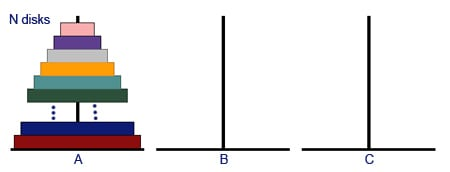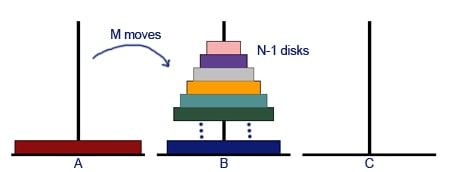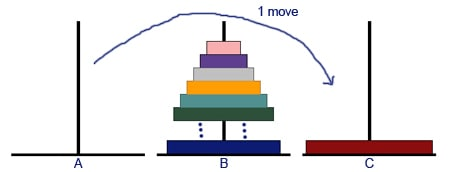The Tower of Hanoi is a famous problem which was posed by a French mathematician in 1883.
What you need to do is move all the disks from the left hand post to the right hand post. You can only move the disks one at a time and you can never place a bigger disk on a smaller disk.

The aim is to try and complete the transfer using the smallest number of moves possible. For example if you have three disks, the minimum number of moves is 7. If you have four disks, the minimum number of moves is 15. Can you complete the problem in the smallest number of moves possible?

Try it out here.

### The minimum number of moves for any number of disks

Many people want to know what the minimum number of moves needed is when you have 5,6,7 or any number of disks.  This can be surprisingly easy to show and will save you time wondering whether you have actually found the most efficient way to solve the Tower of Hanoi problem.

First of all it is easy to show that if you only have one disk it will take only one move.  It is also easy to show that if you have 2 disks the minimum number of moves is 3.  After this, it is possible to show the minimum number of moves using a recursive pattern.

Imagine you know the minimum number of moves for N-1 disks, let us call it M.  It is then possible to show the minimum number of moves for N disks.

 Number of disks Minimum number of moves 1 1 2 3 … … N-1 M N ?For N disks, first of all move N-1 disks to peg B using the minimum M moves.Then move the biggest disk from post A to post C using 1 move.Then move N-1 disks from post B to post C using the minimum M moves.In total you have used 2M+1 moves to solve the problem for N disks.

We can now use the minimum number of moves for the 1 and 2 disk problems to build up a list of minimum numbers of moves.

 Number of disks Minimum number of moves 1 1 2 3 3 (2 X3)+1 = 7 4 (2X7)+1 = 15 5 (2X15)+1=31 6 (2X31)+1=63 … … N-1 M N 2M+1

### Minimum moves with the Tower of Hanoi

Can we see a pattern in the following list of minimum number of moves: 1,3,7,15,31,63,…?

They are actually powers of 2 with one subtracted : 2N-1

So we now have a formula for the minimum moves with the Tower of Hanoi.

In one version of the puzzle Brahmin priests are completing the puzzle with 64 golden disks.

If you had 64 golden disks you would have to use a minimum of 264-1  moves.  If each move took one second, it would take around 585 billion years to complete the puzzle!

 One reason most employers value people with mathematical skills is that they can think logically and break down difficult problems and come up with solutions.  Being able to solve and understand a problem like the Tower of Hanoi shows that you have an analytical mind and are able to problem solve.

Article by Hazel Lewis